# 深入理解Java中的不可变对象以下是本文目录大纲：

https://www.cnblogs.com/dolphin0520/p/10693891.html

## 一.什么是不可变对象

 1 `不可变对象(Immutable Object)：对象一旦被创建后，对象所有的状态及属性在其生命周期内不会发生任何变化。`

 1 2 3 4 5 6 7 8 9 10 11 `public` `class` `ImmutableObject {` `    ``private` `int` `value;` `    ` `    ``public` `ImmutableObject(``int` `value) {` `        ``this``.value = value;` `    ``}` `    ` `    ``public` `int` `getValue() {` `        ``return` `this``.value;` `    ``}` `}`

 1 2 3 4 5 6 7 8 9 10 `public` `class` `Test {` `    ``public` `static` `void` `main(String[] args) {` `        ``String str = ``"I love java"``;` `        ``String str1 = str;` `        ``System.out.println(``"after replace str:"` `+ str.replace(``"java"``, ``"Java"``));` `        ``System.out.println(``"after replace str1:"` `+ str1);` `    ``}` `}`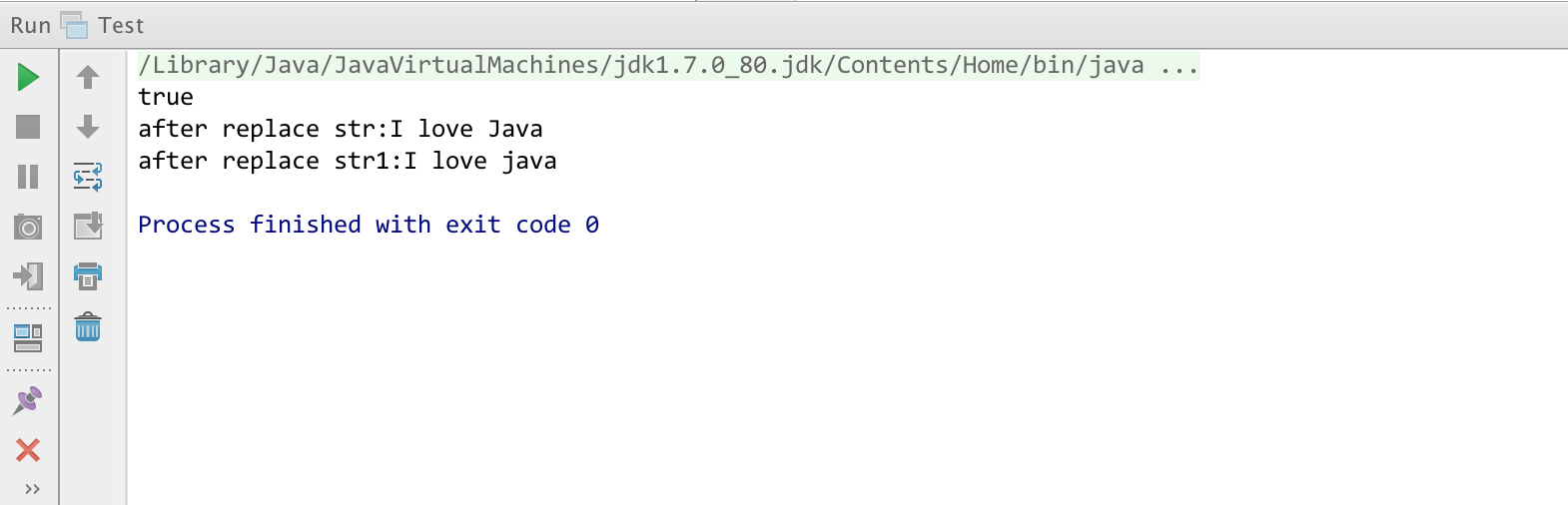## 二.深入理解不可变性

#### 1）让并发编程变得更简单

 1 2 3 4 5 6 7 8 9 10 11 12 13 14 15 16 17 18 19 20 21 22 23 24 25 26 27 28 29 30 31 32 33 34 35 36 37 38 39 `public` `class` `SynchronizedRGB {` `    ``private` `int` `red;  ``// 颜色对应的红色值` `    ``private` `int` `green; ``// 颜色对应的绿色值` `    ``private` `int` `blue;  ``// 颜色对应的蓝色值` `    ``private` `String name; ``// 颜色名称` `    ``private` `void` `check(``int` `red, ``int` `green, ``int` `blue) {` `        ``if` `(red < ``0` `|| red > ``255` `|| green < ``0` `|| green > ``255` `                ``|| blue < ``0` `|| blue > ``255``) {` `            ``throw` `new` `IllegalArgumentException();` `        ``}` `    ``}` `    ``public` `SynchronizedRGB(``int` `red, ``int` `green, ``int` `blue, String name) {` `        ``check(red, green, blue);` `        ``this``.red = red;` `        ``this``.green = green;` `        ``this``.blue = blue;` `        ``this``.name = name;` `    ``}` `    ``public` `void` `set(``int` `red, ``int` `green, ``int` `blue, String name) {` `        ``check(red, green, blue);` `        ``synchronized` `(``this``) {` `            ``this``.red = red;` `            ``this``.green = green;` `            ``this``.blue = blue;` `            ``this``.name = name;` `        ``}` `    ``}` `    ``public` `synchronized` `int` `getRGB() {` `        ``return` `((red << ``16``) | (green << ``8``) | blue);` `    ``}` `    ``public` `synchronized` `String getName() {` `        ``return` `name;` `    ``}` `}`

 1 2 3 `SynchronizedRGB color =  ``new` `SynchronizedRGB(``0``, ``0``, ``0``, ``"Pitch Black"``);` `int` `myColorInt = color.getRGB();      ``// Statement1` `String myColorName = color.getName(); ``// Statement2`

 1 `color.set(``0``, ``255``, ``0``, ``"Green"``);`

 1 2 3 4 `synchronized` `(color) {` `    ``int` `myColorInt = color.getRGB();` `    ``String myColorName = color.getName();` `}`

 1 2 3 4 5 6 7 8 9 10 11 12 13 14 15 16 17 18 19 20 21 22 23 24 25 26 27 28 29 30 31 32 33 `public` `class` `ImmutableRGB {` `    ``private` `int` `red;` `    ``private` `int` `green;` `    ``private` `int` `blue;` `    ``private` `String name;` `    ``private` `void` `check(``int` `red, ``int` `green, ``int` `blue) {` `        ``if` `(red < ``0` `|| red > ``255` `|| green < ``0` `|| green > ``255` `                ``|| blue < ``0` `|| blue > ``255``) {` `            ``throw` `new` `IllegalArgumentException();` `        ``}` `    ``}` `    ``public` `ImmutableRGB(``int` `red, ``int` `green, ``int` `blue, String name) {` `        ``check(red, green, blue);` `        ``this``.red = red;` `        ``this``.green = green;` `        ``this``.blue = blue;` `        ``this``.name = name;` `    ``}` `    ``public` `ImmutableRGB set(``int` `red, ``int` `green, ``int` `blue, String name) {` `        ``return` `new` `ImmutableRGB(red, green, blue, name);` `    ``}` `    ``public` `int` `getRGB() {` `        ``return` `((red << ``16``) | (green << ``8``) | blue);` `    ``}` `    ``public` `String getName() {` `        ``return` `name;` `    ``}` `}`

### 2）消除副作用

 1 2 3 4 5 6 7 8 9 10 11 12 13 14 15 16 17 18 19 20 21 22 23 24 25 26 27 28 29 30 31 32 33 34 35 36 37 38 39 40 41 42 43 44 45 `class` `Person {` `    ``private` `int` `age;   ``// 年龄` `    ``private` `String identityCardID;  ``// 身份证号码` `    ``public` `int` `getAge() {` `        ``return` `age;` `    ``}` `    ``public` `void` `setAge(``int` `age) {` `        ``this``.age = age;` `    ``}` `    ``public` `String getIdentityCardID() {` `        ``return` `identityCardID;` `    ``}` `    ``public` `void` `setIdentityCardID(String identityCardID) {` `        ``this``.identityCardID = identityCardID;` `    ``}` `}` `public` `class` `Test {` `    ``public` `static` `void` `main(String[] args) {` `        ``Person jack = ``new` `Person();` `        ``jack.setAge(``101``);` `        ``jack.setIdentityCardID(``"42118220090315234X"``);` `        ``System.out.println(validAge(jack));` `　　　　` `　　　　``// 后续使用可能没有察觉到jack的age被修改了` `　　　　``// 为后续埋下了不容易察觉的问题` `    ``}` `    ``public` `static` `boolean` `validAge(Person person) {` `        ``if` `(person.getAge() >= ``100``) {` `            ``person.setAge(``100``);  ``// 此处产生了副作用` `            ``return` `false``;` `        ``}` `        ``return` `true``;` `    ``}` `}`

validAge函数本身只是对age大小进行判断，但是在这个函数里面有一个副作用，就是对参数person指向的对象进行了修改，导致在外部的jack指向的对象也发生了变化。

### 3）减少容器使用过程出错的概率

 1 2 3 4 5 6 7 8 9 10 11 12 13 14 15 16 17 18 19 20 21 22 23 24 25 26 27 28 29 30 31 32 33 34 35 36 37 38 39 40 41 42 43 44 45 46 47 48 49 50 51 52 53 54 55 56 `class` `Person {` `    ``private` `int` `age;   ``// 年龄` `    ``private` `String identityCardID;  ``// 身份证号码` `    ``public` `int` `getAge() {` `        ``return` `age;` `    ``}` `    ``public` `void` `setAge(``int` `age) {` `        ``this``.age = age;` `    ``}` `    ``public` `String getIdentityCardID() {` `        ``return` `identityCardID;` `    ``}` `    ``public` `void` `setIdentityCardID(String identityCardID) {` `        ``this``.identityCardID = identityCardID;` `    ``}` `    ``@Override` `    ``public` `boolean` `equals(Object obj) {` `        ``if` `(obj == ``null``) {` `            ``return` `false``;` `        ``}` `        ``if` `(!(obj ``instanceof`  `Person)) {` `            ``return` `false``;` `        ``}` `        ``Person personObj = (Person) obj;` `        ``return` `this``.age == personObj.getAge() && ``this``.identityCardID.equals(personObj.getIdentityCardID());` `    ``}` `    ``@Override` `    ``public` `int` `hashCode() {` `        ``return` `age * ``37` `+ identityCardID.hashCode();` `    ``}` `}` `public` `class` `Test {` `    ``public` `static` `void` `main(String[] args) {` `        ``Person jack = ``new` `Person();` `        ``jack.setAge(``10``);` `        ``jack.setIdentityCardID(``"42118220090315234X"``);` `        ``Set personSet = ``new` `HashSet();` `        ``personSet.add(jack);` `        ``jack.setAge(``11``);` `        ``System.out.println(personSet.contains(jack));` `    ``}` `}`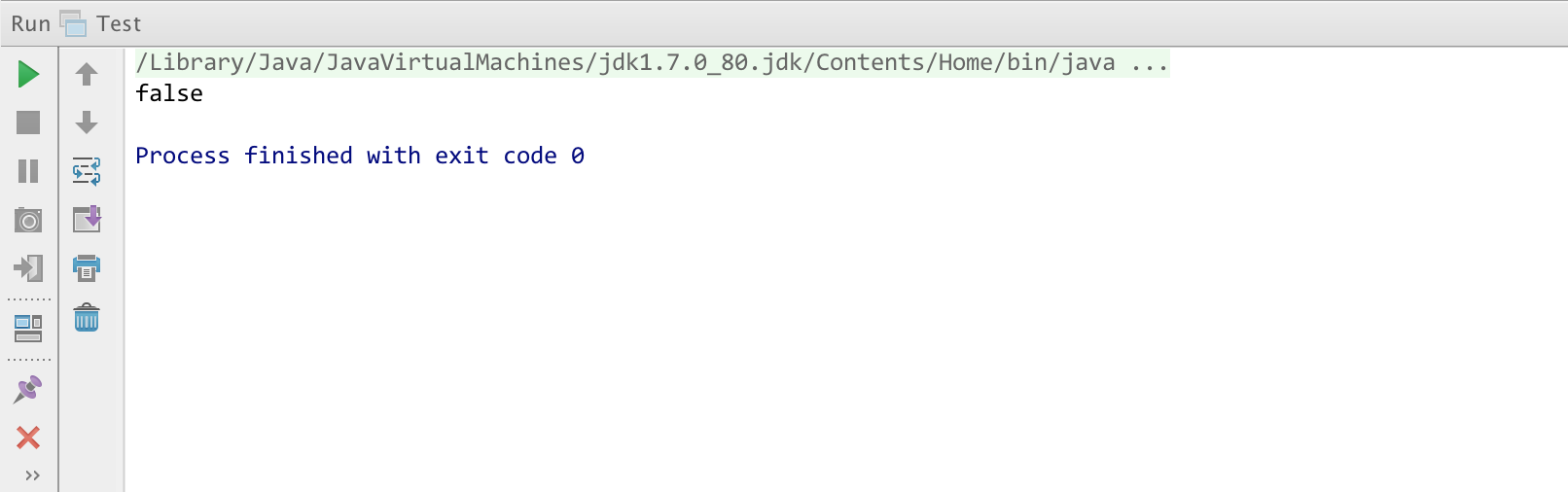## 三.如何创建不可变对象

1）所有成员变量必须是private

2）最好同时用final修饰(非必须)

3）不提供能够修改原有对象状态的方法

• 最常见的方式是不提供setter方法
• 如果提供修改方法，需要新创建一个对象，并在新创建的对象上进行修改

4）通过构造器初始化所有成员变量，引用类型的成员变量必须进行深拷贝(deep copy)

5）getter方法不能对外泄露this引用以及成员变量的引用

6）最好不允许类被继承(非必须)

JDK中提供了一系列方法方便我们创建不可变集合，如：

 1 `Collections.unmodifiableList(List list)`

 1 `ImmutableList.copyOf(list)`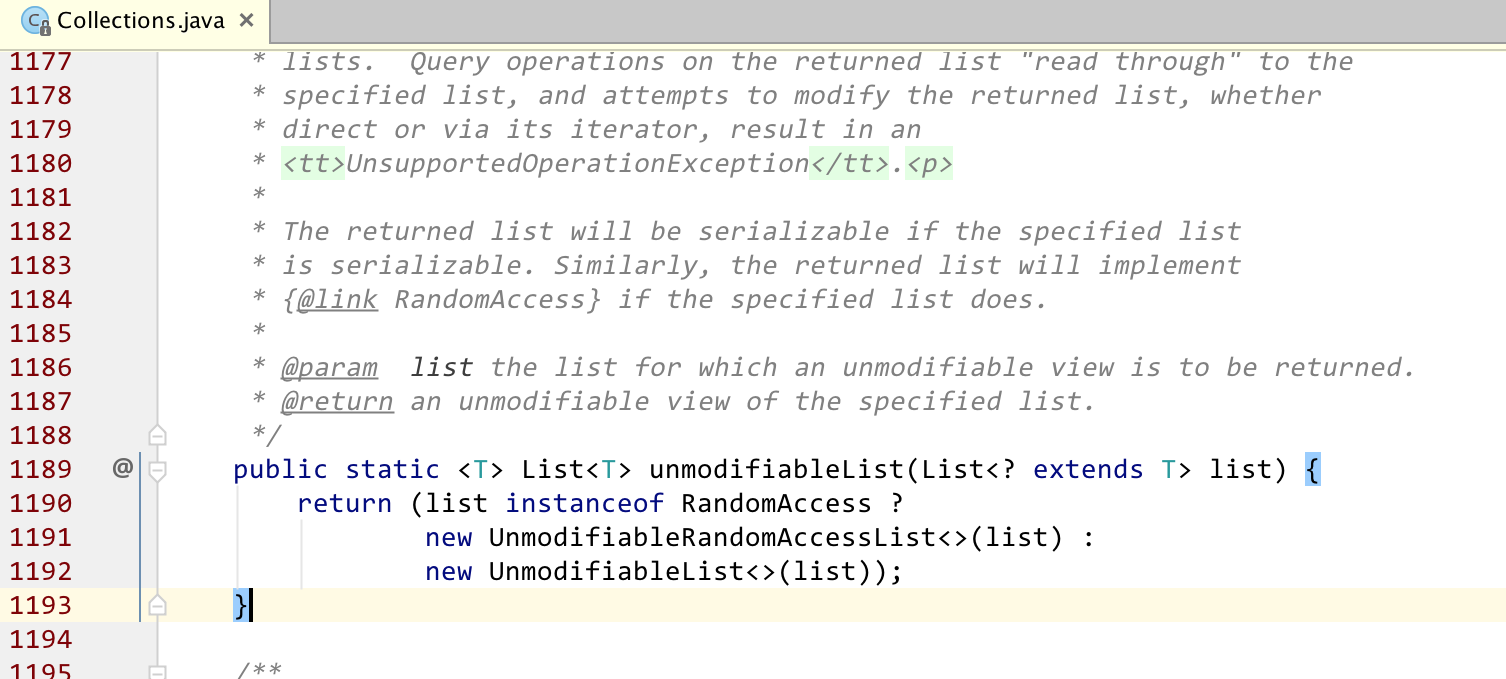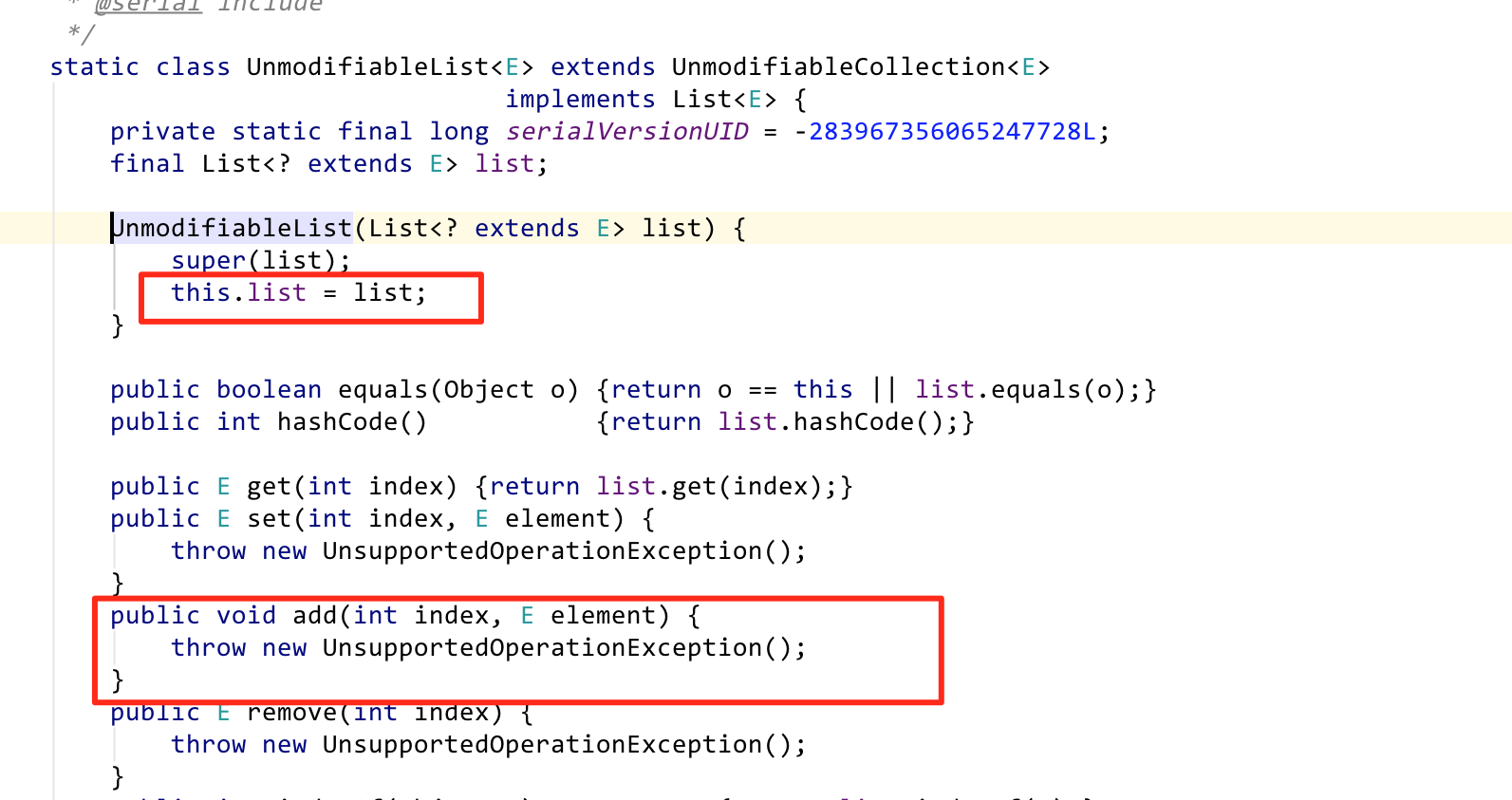1 2 3 4 5 6 7 8 9 10 11 12 13 14 15 16 17 `public` `class` `Test {` `    ``public` `static` `void` `main(String[] args) {` `        ``List list = ``new` `ArrayList();` `        ``list.add(``1``);` `        ``System.out.println(list);` `        ``List unmodifiableList = Collections.unmodifiableList(list);` `        ``ImmutableList immutableList = ImmutableList.copyOf(list);` `        ``list.add(``2``);` `        ``System.out.println(unmodifiableList);` `        ``System.out.println(immutableList);` `    ``}` `}`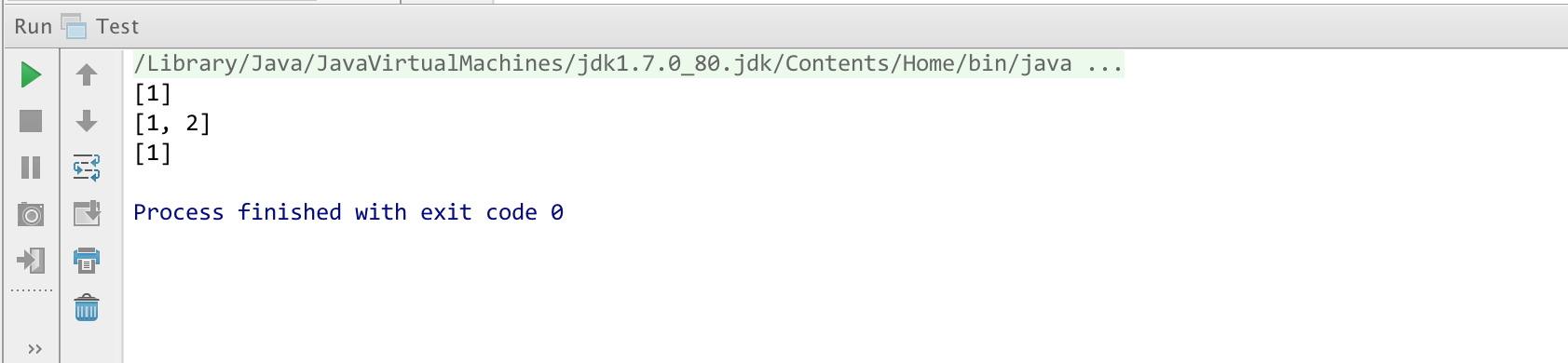## 四.不可变对象真的”完全不可改变”吗？

 1 2 3 4 5 6 7 8 9 10 11 12 13 14 `public` `class` `Test {` `    ``public` `static` `void` `main(String[] args) ``throws` `Exception {` `        ``String s = ``"Hello World"``;` `        ``System.out.println(``"s = "` `+ s);` `        ``Field valueFieldOfString = String.``class``.getDeclaredField(``"value"``);` `        ``valueFieldOfString.setAccessible(``true``);` `        ``char``[] value = (``char``[]) valueFieldOfString.get(s);` `        ``value[``5``] = ``'_'``;` `        ``System.out.println(``"s = "` `+ s);` `    ``}` `}`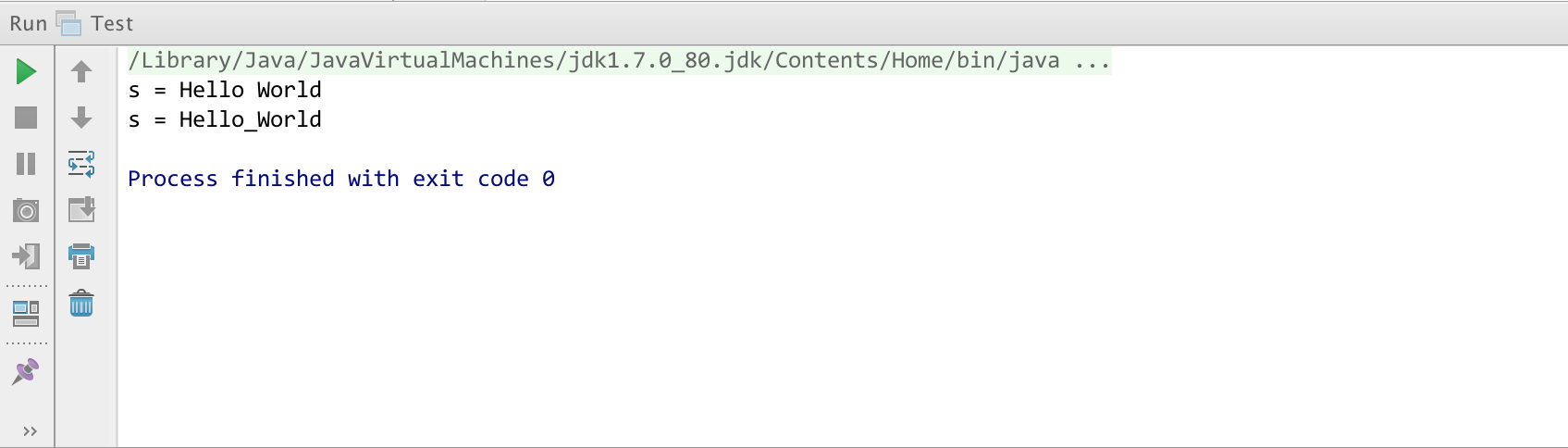http://ifeve.com/immutable-objects/